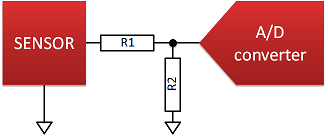# Microcontroller sensor interfacing exercises

MPX6116A pressure sensor
• sensitivity: 45 mV/kPa; offset: 0.2 V at 15 kPa
• output voltage range: 0.2 V to 4.8 V

• Voltage reference: 1.5 V
• Number of bits: 11
• Jusfitication: right

Signal conditioning
• voltage divider
• R1 = 22 k, R2 = 10 kTo do:
• Write a function that calculates the ADC code as a function of the sensed value!
Test data: